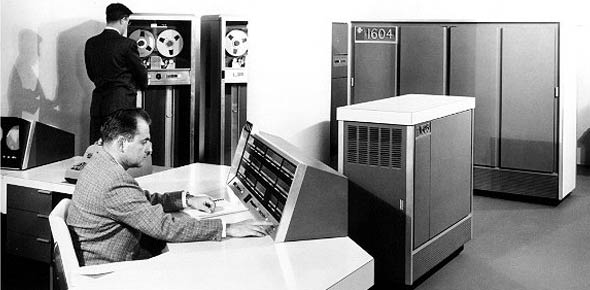# Volume 2 - 2014 2A651 CDC

88 Questions | Attempts: 178Settings• 1.
(201) An aircraft taxiing at a steady speed can be used to demonstrate
• A.

Bernoulli’s principle.

• B.

Newton’s first law of motion.

• C.

Newton’s second law of motion.

• D.

Newton’s third law of motion.

• 2.
(201) Newton’s third law of motion explains why an operating jet engine produces
• A.

Forward thrust.

• B.

Forward acceleration.

• C.

Increased acceleration.

• D.

Decreased acceleration.

• 3.
(201) Which type of duct would decrease the velocity and increase the pressure of a gas as it passes through?
• A.

Elbow.

• B.

Straight.

• C.

Divergent.

• D.

Convergent.

• 4.
(201) "The combination of decreased pressure above an airfoil, and the increased pressure below the airfoil produces lift" is best described by
• A.

Bernoulli’s principle.

• B.

Newton’s first law of motion.

• C.

Newton’s second law of motion.

• D.

Newton’s third law of motion.

• 5.
(202) Foot-pounds and inch-pounds are units of measure for
• A.

Work.

• B.

Power.

• C.

Inertia.

• D.

Friction.

• 6.
(202) What does fuel for an engine represent?
• A.

Available horsepower.

• B.

Thrust produced.

• C.

Potential energy.

• D.

Efficiency ratio.

• 7.
(203) Which section of a jet engine continually supplies air and maintains pressure?
• A.

Turbine.

• B.

Diffuser.

• C.

Compressor.

• D.

Combustion.

• 8.
(203) The temperature of compressed air in a jet engine must be raised to
• A.

Increase energy.

• B.

Decrease energy.

• C.

Increase volume.

• D.

Decrease volume.

• 9.
(203) Which section of a jet engine introduces and burns fuel?
• A.

Turbine.

• B.

Diffuser.

• C.

Compressor.

• D.

Combustion.

• 10.
(204) How many British thermal units (Btu) does the heat content per pound of kerosene produce?
• A.

10,500.

• B.

15,000.

• C.

18,500.

• D.

40,000.

• 11.
(204) The efficiency of a jet engine is often referred to as
• A.

Energy.

• B.

Momentum.

• C.

Thermal efficiency.

• D.

Propulsive efficiency.

• 12.
(204) The product of thermal efficiency and propulsive efficiency is
• A.

Mechanical efficiency

• B.

Overall efficiency

• C.

Power efficiency

• D.

Thrust efficiency

• 13.
(205) To measure barometric pressure, you would use a
• A.

Potentiometer.

• B.

Potentiometer.

• C.

Pyrometer.

• D.

Barometer

• 14.
(205) What effect does moisture content or humidity have on density of air?
• A.

More moisture, more dense

• B.

More moisture, less dense

• C.

Less moisture, less dense

• D.

None.

• 15.
(205) Due to the divergent design of a diffuser, air pressure
• A.

Remains the same

• B.

Fluctuates.

• C.

Decreases.

• D.

Increases.

• 16.
(205) The air temperature of a jet engine gradually rises across the compressor to the diffuser outlet as a result of
• A.

Compression.

• B.

Fuel expansion.

• C.

Turbine discharge.

• D.

Ambient temperature increase.

• 17.
(205) Where is the highest point of temperature reached in an engine?
• A.

Tailpipe.

• B.

Exhaust cone.

• C.

Turbine section.

• D.

Combustion section.

• 18.
(206) Which engine component meters fuel for combustion?
• A.

Pressure and drain (P&D) valve.

• B.

Fuel control.

• C.

Fuel nozzle.

• D.

Fuel pump.

• 19.
(206) What happens to the energy that is absorbed by the turbine wheel?
• A.

50 percent is used for accessories.

• B.

60 percent is used for accessories.

• C.

It is returned to the compressor.

• D.

It operates the anti-ice system.

• 20.
(207) What is the required velocity for air and gases flowing through a jet engine?
• A.

Low velocity at all times.

• B.

Less velocity exiting than entering.

• C.

Equal velocity entering and exiting.

• D.

Greater velocity exiting than entering.

• 21.
(207) The velocity of the stream of air that flows through a jet engine reaches its highest point at the
• A.

Ejector nozzle.

• B.

Combustion section.

• C.

Turbine exhaust cone.

• D.

Turbine stator (nozzle diaphragm).

• 22.
(207) What is the general flow of gases from the combustion chamber?
• A.

At right angles to the rotor shaft.

• B.

Parallel to the axis of the rotor shaft.

• C.

At the same velocity as the rotor shaft.

• D.

In a reverse direction from the rotor shaft.

• 23.
(207) Gases enter the jet-engine’s first-stage turbine wheel blades from the
• A.

• B.

Combustion chamber.

• C.

Turbine stator.

• D.

Flameholder.

• 24.
(207) When two or more turbine wheels are used in a jet engine, which component is placed directly in front of each turbine wheel?
• A.

Diffuser.

• B.

Jet nozzle.

• C.

Combustion chamber.

• D.

Turbine stator (nozzle diaphragm).

• 25.
(208) A jet engine derives its name from its design in that it uses
• A.

An exhaust-gas-driven turbine wheel to drive its compressor.

• B.

Nozzles which are called jet within the engine.

• C.

A turbine-type compressor to maintain power.

• D.

Turbo-superchargers within the engine.

## Related TopicsBack to top
×

Wait!
Here's an interesting quiz for you.